UPSC  >  Dice: Solved Examples

# Dice: Solved Examples - CSAT Preparation - UPSC

Q.1. A dice with six faces is marked with six numbers 1, 2, 3, 4, 5  and 6 respectively. This dice is rolled three times and three positions are shown as: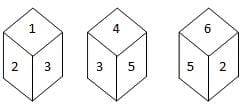Find the number opposite to 1.
A. 2
B. 6
C. 5
D. 4
Explanation:

From figures (ii) and (iii), we can conclude that the numbers 2, 4, 3, and 6 appear adjacent to the letter 5. Therefore, the number 1 appears opposite to 5. In other words, 5 appears opposite to 1.

Q.2. You are given three positions of dice then which face is opposite to the face with alphabet B?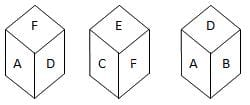A. E
B. F
C. D
D. A
Explanation:
From figures (i) and (ii), we can conclude that the alphabets C, D, A and E lie adjacent to the alphabet F. So, the alphabet B lies opposite F and conversely F lies opposite B.

Q.3. Which face is opposite to face with alphabet B, if four positions are given as below as?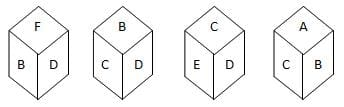A. B
B. A
C. F
D. E
Explanation:
From figures (i), (ii) and (iv), we can conclude that F, D, C and A lie adjacent to B. Hence, E must lie opposite to B.

Q.4. Which of the following patterns can be formed from the piece of cardboard (X) as shown below?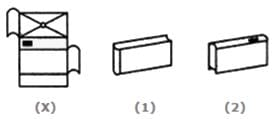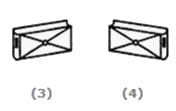A. 4
B. 2
C. 1
D. 3

Explanation:

The pattern on the fig. (X) and the fact that the faces are rectangular and fig.(2) is eliminated as dot on the upper face is on left side instead of right.
Fig. (3) and fig.(4) also get eliminated as the smaller rectangular faces should be blank instead of having a dot on them.
So, fig.(1) is the only possible fig as it is having three blank faces adjacent to each other.

Directions for questions 5 to 9: Six different positions of dice are given below: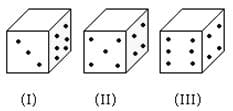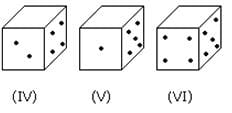The sum of the numbers of dots on the opposite face is 7.

Q.5. If an even numbered dice have an odd number of dots on their top faces, then find the total number of dots on the top faces of their dice?
A. 22
B. 3
C. 10
D. 24
Explanation:

Even numbered dice are: (II), (IV) and (VI)
Number of dots on the top face of (II) dice = 1
Number of dots on the top face of (IV) dice = 1
Number of dots on the top face of (VI) dice = 1
Therefore, the required total = 1+1+1=3

Q.6. If an odd numbered dice have an odd number of dots on their top faces, then find the total number of dots on top faces of their dice is?
A. 11
B. 12
C. 13
D. 14
Explanation:

Odd numbered dice are : (II), (III) and (V)
Numbers of dots on the top faces of these dice are 5, 5 and 3 respectively.
Required total = 5+5+3=13|

Q.7. . If dice (I), (II) and (III) have an odd number of dots on their top faces, and the dice (IV), (V) and (VI) have an even number of dots on their top faces, then what is the difference in the total number of top faces between the two sets?
A. 0
B. 3
C. 1
D. -5
Explanation:

Number of faces on the top faces of the dice (I), (II) and (III) are 5, 1 and 5 respectively.
Therefore, the total of these numbers = 5 + 1 + 5 = 11
Number of dots on the top faces of the dice (IV), (V) and (VI) are 6, 4 and 6 respectively.

Therefore, the total of these numbers = 6+4+6=16
Required difference = 11 -16=-5

Q8. If the odd numbers of dice have an even number of dots on their top faces and an even numbered dice have an odd of dots on their bottom faces, then what is the total number of dots on their top faces?
A. 26
B. 12
C. 16
D. 18
Explanation:

Number of dots on the top faces of the dice (II), (IV) and (VI) are 2, 2 and 4 respectively.
Number of dots on the top faces of the dice (I), (III) and (V) are 6, 6 and 6 respectively.
Required total = 2 + 2 + 4 + 6 + 6 + 6 =26

Q9. If the dice (I), (II) and (III) have an odd number of dots on their top faces, then what is the total number of dots on their top faces?
A. 7
B. 14
C. 12
D. 11
Explanation:

No. of dots on the top faces of dice (I), (II) and (III) are 5, 1 and 5 respectively.
Required total = 5 + 1 + 5 = 11

Q10.Refer to the following four positions of the dice and find out the color which is opposite the face grey?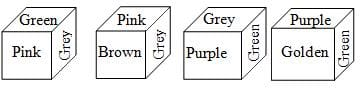A. Golden
B. Purple
C. Brown
D. Green
Explanation

The colors adjacent to grey are green, pink, purple and brown. Hence golden will be opposite to grey.

The document Dice: Solved Examples | CSAT Preparation - UPSC is a part of the UPSC Course CSAT Preparation.
All you need of UPSC at this link: UPSC

## CSAT Preparation

197 videos|151 docs|200 tests

## FAQs on Dice: Solved Examples - CSAT Preparation - UPSC

 1. What are dice used for in games?Ans. Dice are small objects with different numbers or symbols on each side. They are commonly used in games as a way to introduce an element of chance or randomness. Players roll the dice and the outcome determines their next move or action in the game.
 2. How many sides does a standard dice have?Ans. A standard dice typically has six sides, with each side having a different number of dots or pips ranging from 1 to 6. This type of dice is commonly used in various board games and gambling activities.
 3. Are there dice with more than six sides?Ans. Yes, there are dice with more than six sides. These dice are known as polyhedral dice and are commonly used in role-playing games such as Dungeons & Dragons. Polyhedral dice can have various shapes, including 4-sided (tetrahedron), 8-sided (octahedron), 10-sided (decahedron), 12-sided (dodecahedron), and 20-sided (icosahedron) dice.
 4. How do dice ensure fairness in games?Ans. Dice are designed to provide a random outcome, ensuring fairness in games. The numbers or symbols on each side of the dice are evenly distributed, and the shape and weight of the dice are carefully balanced. When rolled, the dice have an equal probability of landing on any side, minimizing any bias or unfair advantage.
 5. Can dice be used for educational purposes?Ans. Yes, dice can be used for educational purposes. Teachers often use dice in various educational activities and games to make learning more engaging and interactive. Dice can be used for math games, vocabulary building, probability experiments, and decision-making exercises, among other educational applications.

## CSAT Preparation

197 videos|151 docs|200 testsExplore Courses for UPSC exam### How to Prepare for UPSC

Read our guide to prepare for UPSC which is created by Toppers & the best Teachers
Signup to see your scores go up within 7 days! Learn & Practice with 1000+ FREE Notes, Videos & Tests.
10M+ students study on EduRev
Track your progress, build streaks, highlight & save important lessons and more!
Related Searches

,

,

,

,

,

,

,

,

,

,

,

,

,

,

,

,

,

,

,

,

,

;